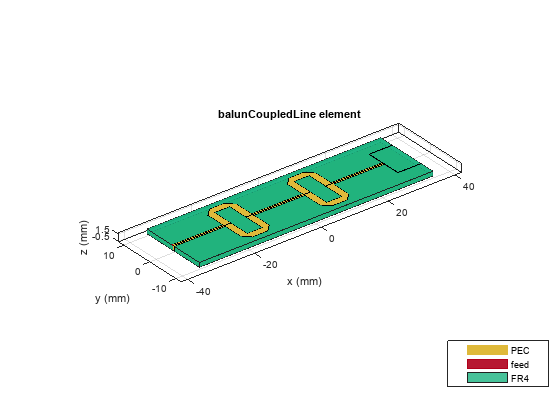# designOutputLine

Calculate dimensions of output line section for specified frequency

## Syntax

``[Length,Width] = designOutputLine(balunobj,frequency)``
``___ = designOutputLine(___,Name=Value)``

## Description

````[Length,Width] = designOutputLine(balunobj,frequency)` calculates the dimensions of the output line section of a coupled-line balun around a specified frequency. Note`designOutputLine` is the third step in designing a coupled line balun. This function is preceded by `designCoupledLine` and `designUncoupledLine` as the first and second step, respectively. ```

example

````___ = designOutputLine(___,Name=Value)` calculates the dimensions of the output line section of a coupled-line balun with additional options specified using name-value arguments. NotePCB components designed using the `design` function operate around the specified frequency with a 10-15% tolerance. ```

## Examples

collapse all

Define the frequency at 4 GHz.

`f = 4e9;`

Create a coupled line balun object.

`balun = balunCoupledLine`
```balun = balunCoupledLine with properties: NumCoupledLineSection: 3 CoupledLineLength: 0.0153 CoupledLineWidth: 4.0000e-04 CoupledLineSpacing: 1.4000e-04 UncoupledLineShape: [1×1 ubendMitered] OutputLineLength: 0.0124 OutputLineWidth: 1.5300e-04 OutputLineSpacing: 0.0110 Height: 0.0013 GroundPlaneWidth: 0.0200 Substrate: [1×1 dielectric] Conductor: [1×1 metal] ```
`show(balun)`Step 1: Design coupled line section

Design the coupled line section of the balun with an even mode impedance of 159 ohms and an odd mode impedance of 51 ohms. Use the helper function designCoupledLine.

`[ClineL,ClineW,ClineS] = designCoupledLine(balun,f,'Z0e',159,'Z0o',51)`
```ClineL = 0.0107 ```
```ClineW = 4.2682e-04 ```
```ClineS = 1.4374e-04 ```

Step 2: Design uncoupled line section

Design the uncoupled line section of the balun with the even and odd mode impedance of 59 ohms. Use the helper function designUncoupledLine.

`[unclineL,unclineW] = designUncoupledLine(balun,f,'Z0',59,'LineLength',0.25)`
```unclineL = 0.0103 ```
```unclineW = 0.0018 ```

Step 3: Design output line section

Design the output line section of the balun at the same frequency to extend the port 2 and port3. Use the helper function designOutputLine.

`[OutL,OutW] = designOutputLine(balun,f,'Z0e',159,'Z0o',51,'Z0',59,'Zref',50)`
```OutL = 0.0109 ```
```OutW = 1.6115e-04 ```

Set all the design dimensions to the coupled balun object.

```balun.CoupledLineLength = ClineL; balun.CoupledLineWidth = ClineW; balun.CoupledLineSpacing = ClineS; UnCoupledLine = ubendMitered; UnCoupledLine.Length = [unclineL/2,unclineL/4,unclineL/2]; UnCoupledLine.Width = [unclineW,unclineW,unclineW]; balun.UncoupledLineShape = UnCoupledLine; balun.OutputLineLength = OutL; balun.OutputLineWidth = OutW; balun.OutputLineSpacing = OutL+ClineS; gndW = 25e-3; balun.GroundPlaneWidth = gndW; show(balun)```Analyze and plot the S-paramters of this balun.

```s11 = sparameters(balun,linspace(3.5e9,4.5e9,31)); figure; rfplot(s11,1,1); hold on; rfplot(s11,1,3) hold on; rfplot(s11,1,2)```## Input Arguments

collapse all

Coupled-line balun, specified as a `balunCoupledLine` object.

Example: ```balunobj = balunCoupledLine; design(balunobj,2e9)``` designs a coupled-line balun around a frequency of 2 GHz and returns the OutputLineLength andOuputLineWidth properties of the coupled line balun.

Design frequency of coupled-line balun, specified as a real positive scalar in hertz.

Example: `55e6`

Data Types: `double`

### Name-Value Arguments

Example: `Z0o=61`

Even mode impedance of the coupled-line, specified as a positive scalar in ohms.

Note

The even mode impedance should be same as the even mode impedance of the coupled line section.

Data Types: `double`

Odd mode impedance of the coupled-line, specified as a positive scalar in ohms.

Note

The odd mode impedance should be same as the odd mode impedance of the coupled line section.

Data Types: `double`

Impedance of the uncoupled-line, specified as a positive scalar in ohms.

Note

The impedance should be same as the impedance of the uncoupled line section.

Data Types: `double`

Reference impedance to match the output, specified as a positive scalar in ohms.

Data Types: `double`

## Output Arguments

collapse all

Length of the output line section, returned as a positive scalar.

Width of the output line section, returned as a positive scalar.

## Version History

Introduced in R2022a